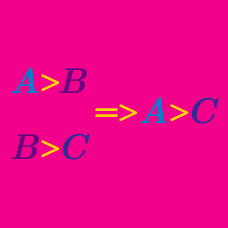Logic

Logical Reasoning

Challenge Quizzes

Think of a number. Multiply it by 2 and add 4. Multiply the result by 0.5 and add 3, and then subtract the original number. I know what number you have left. What must it be?

Think of a positive integer. Multiply it by 5 and add 2. Multiply the result by 2 and subtract 7. I know the last digit of the number you have now. What is it?

Think of any integer between 1 through 9 inclusive. Multiply it by 2 and add 1. Then multiply the result by 5 and add 3. I know the last digit of the number you ended up with. What is it?

Think of a number. Multiply it by 3 and subtract 1. Divide that by 0.5 and add 7. Subtract 6 times the original number. I know what number you have left. What must it be?

I am thinking of a whole number. I multiply it by 4 and add 6. Then I multiply the result by 0.5 and add 2, to end up with 9. Can you guess the number I first thought of?

×